Mobile QR Code1. (Electrical Engineering College, Henan University of Science and Technology, Luoyang 471023, China)

PSO-ELM neural network algorithm, small-signal model, optimization, InP HBT

## I. INTRODUCTION

Heterojunction bipolar transistors (HBTs) have a wide range of applications in microwave circuits due to wide bandgap, high current gain and high cutoff frequency [1-3]. However, HBTs have higher turn-on voltage than Si-based devices. The increase of power dissipation leads to the increase of the junction temperature, which can have a significant impact on the main properties of the devices [4-7]. In order to efficiently use HBTs in computer-aided design, a stable and accurate HBT small-signal model is indispensable.

In the previous HBT modeling works [8,9], many techniques have been proposed, such as directly extracting the equivalent circuit model parameters, using related algorithms to optimize some model parameters. The direct extraction method is completely composed of a set of closed-loop formulas, but when the high-accuracy model is essential, parameters extraction process will be very complicated and time-consuming [9-13]. While the related algorithms are adopted to optimize model parameters, additional formulas are still needed for calculation, and multiple optimizations are required to obtain the optimal parameters . The neural network has been proved to be a good choice for HBT modeling. In , a behavior model based on BP neural network algorithm was proposed. In the process of learning, relevant parameters of neural network are fine-tuned to establish a small signal model. However, the determination of initial network weights has a direct influence on the fitting effect of BP neural network. Different weights will make obvious differences in modeling effect.

In , the Neuro-Space method was adopted for the modeling of HBT. The established model is accurate, yet the training process is not enough simple, since it is necessary to map the fine model on the basis of the establishment of the coarse model for the training process. In , a Wiener-type dynamic neural network is used for modeling. The new training algorithm has good accuracy and applicability. However, the model does not show a relatively good fitting effect under the high-frequency condition of S-parameters. In the study of HEMT models, the Particle Swarm Optimization (PSO) algorithm has been used to model the device characteristics, which shows excellent modelling effects, but they still have the possibility of falling into local optimal solutions [18,19]. In order to solve this problem, the Extreme Learning Machine (ELM) algorithm is proposed to optimize the PSO algorithm for modelling HBT characteristics.

The ELM is a single hidden layer feedforward neural network algorithm . It has fast learning speed and good generalization performance. And it only needs to set the number of hidden nodes in the network to generate the optimal solution. The ELM has been applied in flood prediction, battery management, material analysis, intelligent recognition and other fields [21-24], but it has not been used in small-signal model of HBTs. However, random input weights and hidden layer thresholds may lead to ill-conditioned problems. The PSO is used to optimize the input weights and hidden deviations, which greatly improves the convergence ability of the ELM. In this paper, PSO-ELM algorithm is used to model the small-signal behavior of InP HBT.

The structure of this paper is as follows. In section 2, the InP HBT small-signal equivalent circuit is introduced. In section 3, the PSO-ELM algorithm are proposed. In section 4, the feasibility of PSO-ELM algorithm applied to InP HBT DC and small-signal model is verified. In section 5, the article is summarized.

## II. SMALL-SIGNAL EQUIVALENT CIRCUIT

The InP HBT small-signal equivalent circuit is composed of intrinsic and extrinsic elements. In the intrinsic part, R$_{\mathrm{bi}}$ is the intrinsic base resistance, C$_{\mathrm{bc}}$ is the base-collector capacitance, R$_{\mathrm{be}}$ is the base-emitter resistance, C$_{\mathrm{be}}$ is the base-emitter capacitance, R$_{\mathrm{bc}}$ is the base-collector resistance, and ${\alpha}$ is the common-base current amplification factor. In the extrinsic circuit model, L$_{\mathrm{b}}$, L$_{\mathrm{c}}$, and L$_{\mathrm{e}}$ are the base, collector, and emitter pad parasitic inductances, respectively, C$_{\mathrm{pbc}}$, C$_{\mathrm{pbe}}$, and C$_{\mathrm{pce}}$ are the pad parasitic inductances. R$_{\mathrm{b}}$, R$_{\mathrm{c}}$, and R$_{\mathrm{e}}$ are the extrinsic base, collector, and emitter resistances, respectively. C$_{\mathrm{bcx}}$ is the extrinsic base-collector capacitance .

## III. EXTREME LEARNING MACHINE

The ELM is a single hidden layer feedforward neural network algorithm . Instead of traditional gradient-based feedforward network learning algorithms [27,28], ELM uses random input layer weights and hidden layer biases, making it faster to compute. As shown in Fig. 4. The ELM model uses a multilayer perceptron structure, which consists of three layers of neurons. The input layer is Frequency, collector-emitter voltage (V$_{\mathrm{ce}}$) and collector current (I$_{\mathrm{c}}$), the middle hidden layer includes neurons, and the right output layer are S-parameters.

The number of neurons in the hidden layer depends on the complexity of the problem. The number will significantly affect the training and prediction results of ELM. In this paper, we used trial and error method to determine the number of hidden layer neuron nodes, and 46,48,50,52,54,56,58 hidden layer neurons in ELM and PSO-ELM models are set for training and testing. The relationship between the mean square error of training sample and the number of hidden layer is shown in Fig. 2, and the relationship between the mean square error of prediction sample and the number of hidden layer is shown in Fig. 3. It can be seen that, with the increase of the number of hidden layer nodes, the training error will gradually decrease and tend to approach zero, and the prediction error will increase significantly with the change of the number of hidden layer, which is due to the over-fitting error. Hence, the number of hidden layer neurons in this paper is determined to be 50. In this experiment, there are 3 input samples, 50 hidden node neurons, and 8 output layer parameters. The mathematical derivation process of this experiment is shown in Eqs. (1)-(11). g(x) is the activation function, using the sigmoid function. ${\omega}$$_{\mathrm{ij}} is the randomly generated input weight connecting the i-th (i=1, 2, 3, …, 50) hidden layer and the j-th (j=1, 2, 3) input layer. b_{\mathrm{i}} is the deviation of the i-th node of the hidden layer. {\beta}$$_{\mathrm{ij}}$is the output weight connecting the i-th hidden layer and the j-th output layer. The input weight ${\omega}$, hidden bias b, and output weight ${\beta}$ can be obtained from Fig. 2, as shown in Eqs. (1)-(3). h$_{\mathrm{i}}$(x) is the output matrix of the hidden layer, which is calculated by a strict mathematical formula from the input weight and the hidden layer deviation. T is the target matrix of training data.

##### Fig. 1. Small-Signal Model of InP HBT.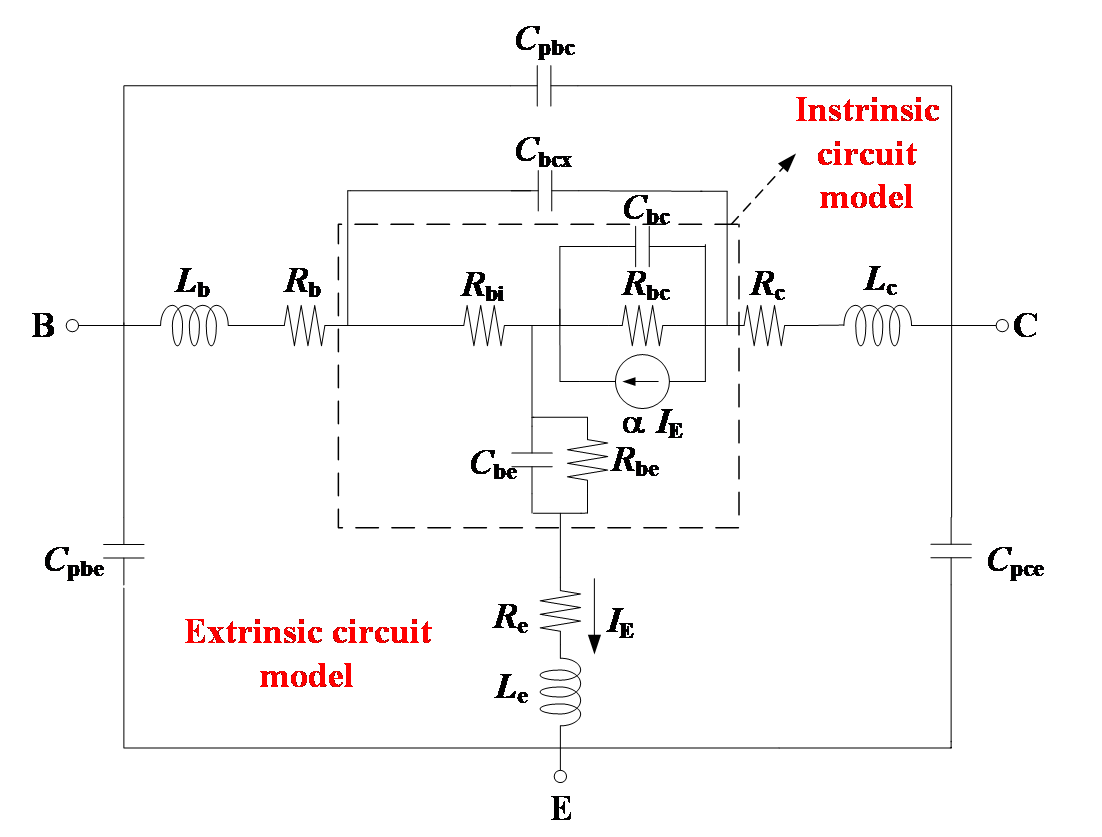##### Fig. 2. The relationship between mean square error of training sample and number of hidden layer neurons.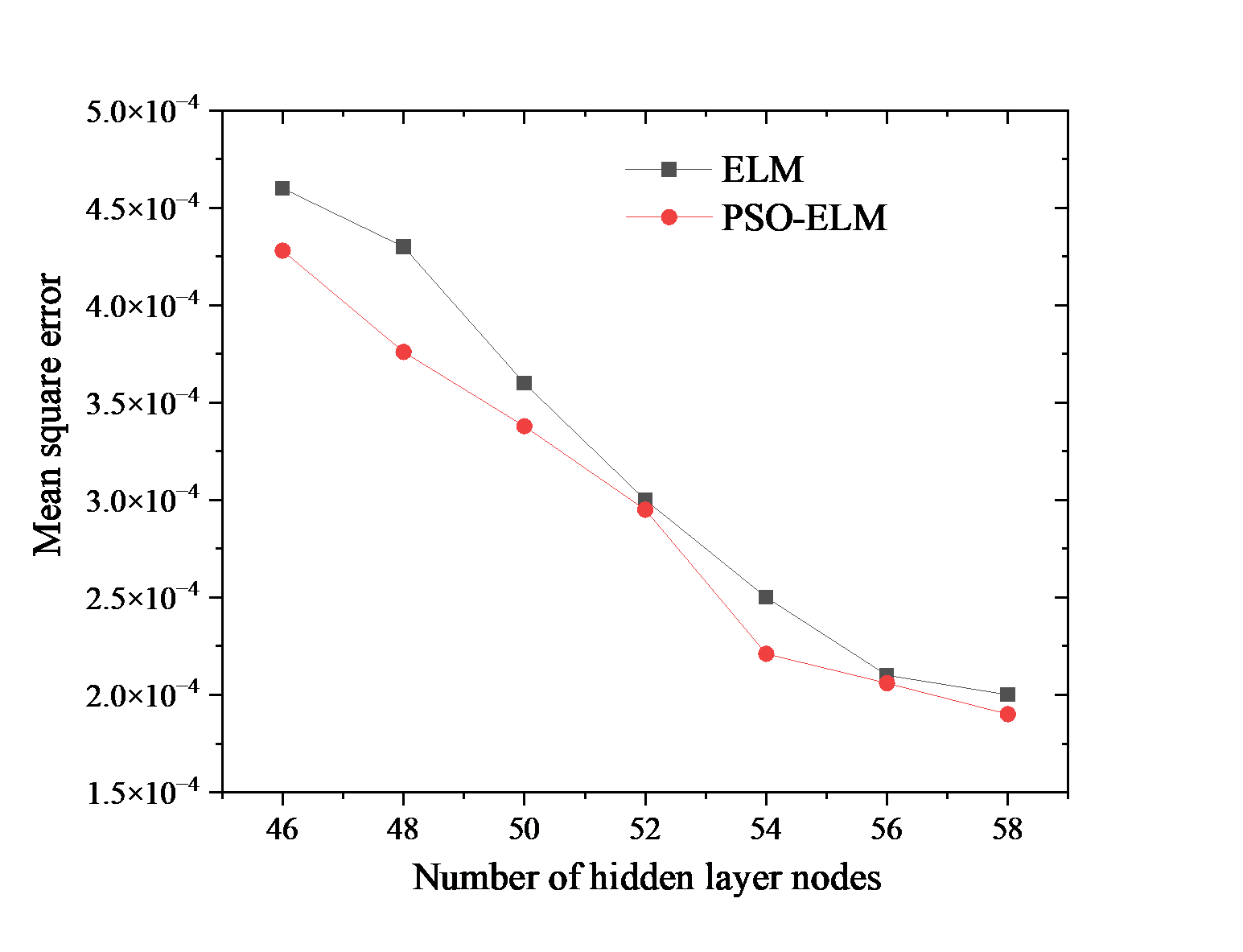##### (1)
$b_{\mathrm{i}}=\left[b_{1},b_{2},\cdots ,b_{\mathrm{i}}\right]^{\mathrm{T}} \\$
##### (2)
$\omega _{\mathrm{i}}=\left[\omega _{\mathrm{i}1},\omega _{\mathrm{i}2},\omega _{\mathrm{i}3}\right]^{\mathrm{T}} \\$
##### (3)
$\beta _{\mathrm{i}}=\left[\beta _{\mathrm{i}1},\cdots \beta _{\mathrm{i}8}\right]^{\mathrm{T}} \\$
##### (4)
$h_{i}(x)=g(\omega _{i},b_{i},x)=g(\omega _{i}x+b_{i}) \\$
##### (5)
$$H=\left[h\left(x_1\right), \ldots h\left(x_{\mathrm{i}}\right)\right]^{\mathrm{T}}=\left[\begin{array}{ccc} h_1\left(x_1\right) & \cdots & h_{50}\left(x_1\right) \\ \vdots & \vdots & \vdots \\ h_1\left(x_{50}\right) & \cdots & h_{50}\left(x_{50}\right) \end{array}\right]$$
##### (6)
$T=\left[\begin{array}{l} {t_{1}}^{T}\\ \vdots \\ {t_{50}}^{T} \end{array}\right] \\$
##### (7)
$H\cdot \beta =T$

The output weight ${\beta}$ can be calculated by the following formula, where H$^{+}$ is the Moore-Penrose generalized inverse matrix of matrix H .

##### (8)
$\beta =H^{+}T=\left(H^{T}H\right)^{-1}H^{T}T$

### 1. Particle Swarm Optimization Algorithm

The PSO algorithm was proposed by Kennedy and Eberhart . It achieves the purpose of searching for the global optimal solution by modeling and simulating the social behavior of birds or fish groups. All particles are randomly initialized and fly at a certain speed. In each iteration, according to the influence of its own historical best position and the best position of adjacent particles, its velocity vector is adjusted to obtain the global optimal solution. The velocity of each particle is updated according to Eq. (9).

##### (9)
$v\mathrm{id}\left(\mathrm{t}+1\right)=\omega \cdot v\mathrm{id}\left(\mathrm{t}\right)+c1\cdot rand\left(0,1\right)\cdot \left[p\mathrm{id}\left(\mathrm{t}\right)-x\mathrm{id}\left(\mathrm{t}\right)\right]\\ +c2\cdot rand\left(0,1\right)\cdot \left[p\mathrm{gd}\left(\mathrm{t}\right)-x\mathrm{id}\left(\mathrm{t}\right)\right]$

where c$_{1}$ is the individual learning factor of each particle, and c$_{2}$ is the social learning factor of each particle. p$_{\mathrm{id}}$ represents the d-th dimension of the individual extreme value of the i-th variable, and p$_{\mathrm{gd}}$ represents the d-th dimension of the global optimal solution. rand(0,1) is represented as a random number between 0-1. v$_{\mathrm{id}}$(t+1) is the velocity of the i-th particle after the update of the d-th dimension, and v$_{\mathrm{id}}$ is the velocity of the i-th particle before the update of the d-th dimension.

Eq. (10) shows the position of each particle which has been updated after iteration. The maximum velocity of v$_{\mathrm{id}}$ is v$_{\mathrm{max}}$. x$_{\mathrm{id}}$(t+1) is the position of the i particle in the d-th dimension after the update, and x$_{\mathrm{id}}$(t) is the position of the i-particle in the d-th dimension before the update. In order to obtain better optimization effect, Eq. (11) was adopted. ${\omega}$ is the new inertia weight, which gradually decreases as the number of iterations increases. ${\omega}$$_{\mathrm{max}} is the maximum inertia weight. {\omega}$$_{\mathrm{min}}$ is the minimum inertia weight. t$_{\mathrm{max}}$ is the maximum number of iterations, and t is the current number of iterations. The size of the inertia weight will affect the quality of the global optimization. Therefore, the use of dynamic inertia factor can obtain better optimization results.

##### (10)
$x_{\mathrm{id}}(t+1)=x_{\mathrm{id}}(t)+v_{\mathrm{id}}(t+1) \\$
##### (11)
$\omega =\omega _{\max }-\frac{\omega _{\max }-\omega _{\min }}{t_{\max }}\cdot t$

### 2. Extreme Learning Machine based on Particle Swarm Optimization

ELM has very fast calculation speed due to its randomly determined input layer weights and hidden layer biases of single-hidden layer feedforward neural network, and it still retains the general approximation ability of single-hidden layer feedforward neural network while randomly generating hidden layer nodes, so it also has the advantages of less training parameters and strong generalization ability. However, to model the InP HBT small-signal behavior, the number of samples in the training set is large, and when more hidden layer neurons are required, the response speed and accuracy of the ELM to the test data may be affected. Computed from randomly determined input layer weights and hidden layer biases, randomly chosen parameters may result in ill-conditioned output matrices and poor generalization . Therefore, in this paper the input weights and hidden deviations are optimized by the PSO, so as to achieve the purpose of improving the accuracy of the InP HBT neural network model. The specific steps of the PSO-ELM algorithm proposed in this paper are as follows, and the algorithm flowchart is shown in Fig. 3.

(1) The data is divided into training set and test set, then the number of neurons in the hidden layer is set and the activation function is selected.

(2) When the PSO is initialized, the maximum number of iterations and the number of populations are set to be 300 and 40 respectively. Moreover, the example speed range is -6~6, and the inertia weight is 0.2~0.9.

(3) The input weights and hidden layer deviations are taken as part of each particle in the particle swarm. The positions and velocities of the particles are adjusted while the ELM is trained, and the mean squared error is calculated. The Mean Squared Error (MSE) expressed as Eq. (12) is used to quantify the agreement between the measured and modeled results. The MSE is also used as the fitness function, which is expressed as the absolute value of the error between the test set and the training value.

(4) The individual and global extreme values are updated according to calculated particles fitness, and it is judged whether the minimum fitness is reached. If it is reached, the particles containing the input weights and hidden layer thresholds are output to the ELM related model, otherwise it returns to continue to adjust the position of the particles with speed.

(5) According to the optimal weight and threshold of the output, the output matrix of the hidden layer is calculated. The output matrix and output weights are used to verify the InP HBT small-signal model established in this paper.

##### (12)
$MSE=\sum _{\mathrm{j}=1}^{\mathrm{N}}\left| \sum _{\mathrm{i}=1}^{\mathrm{N}}\beta _{\mathrm{i}}\cdot g\left(\omega _{\mathrm{i}}x+b_{\mathrm{i}}\right)-T_{\text{test}}\right|$
##### Fig. 3. The relationship between mean square error of prediction sample and number of hidden layer neurons.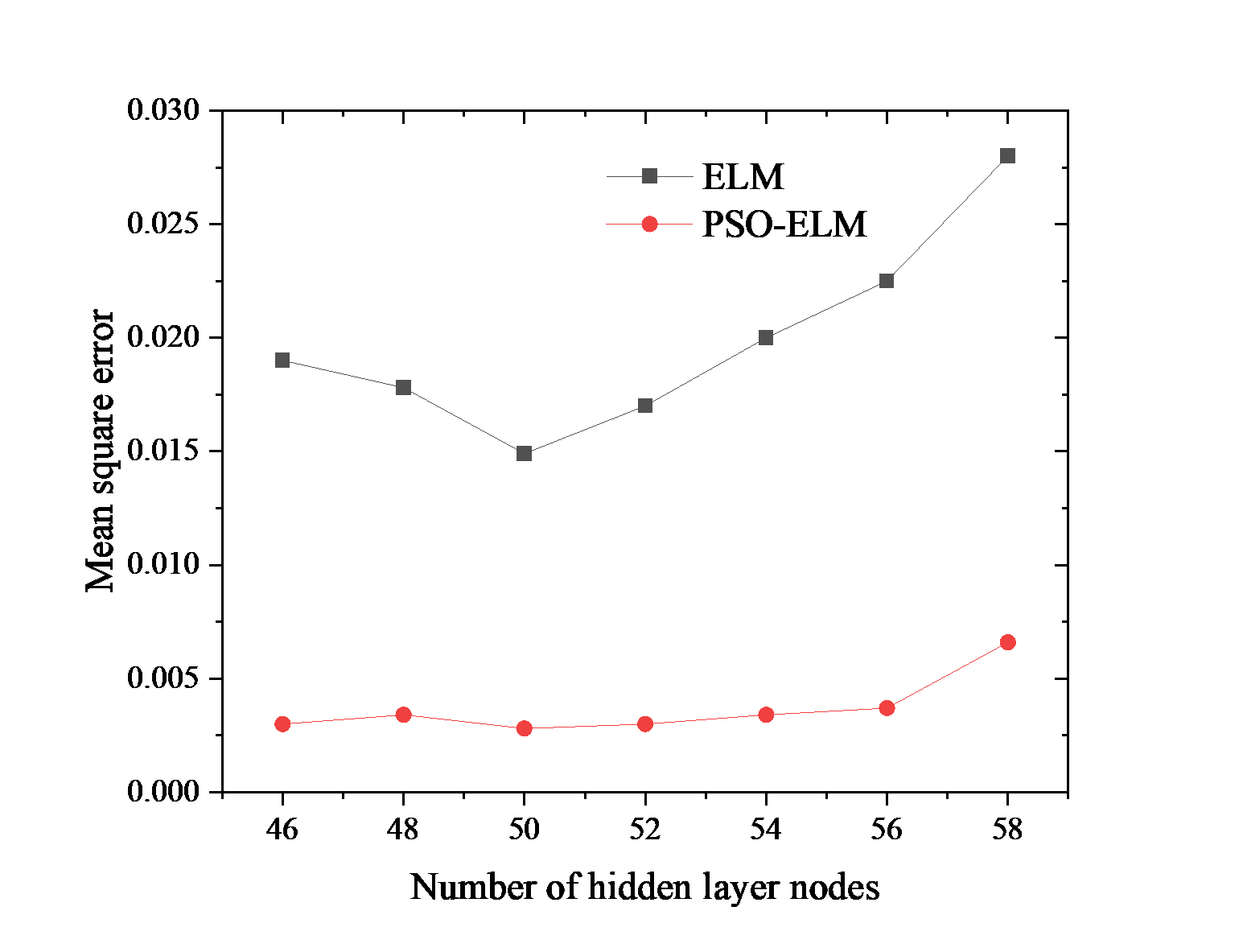## IV. RESULTS AND DISCUSSION

In this paper, InP HBT devices are used to verify the accuracy of the small-signal model. It was implemented by the Institute of Microelectronics, Chinese Academy of Sciences, with an emitter area of 1 ${\mu}$m${\times}$15 ${\mu}$m. The Agilent 8510C Vector Network Analyzer was used to test the S-parameters, and the Agilent B1500A Semiconductor Device Analyzer provided the DC bias for the DUT. The test process is controlled by IC-CAP software, and the S-parameters in the frequency range of 0.1 ~ 40 GHz are measured and obtained. All test processes are on-chip performed. The algorithm is tested on the i7-10710U processor.

The modeling results of I$_{\mathrm{c}}$-V$_{\mathrm{ce}}$ characteristics are shown in Fig. 6. It can be found that all three models can effectively fit the measured data. But with the increase of I$_{\mathrm{B}}$ value, the fitting effect of BP model has a large deviation. The fitting effect of ELM model is better than that of BP model. The PSO-ELM model has good fitting effect and can accurately predict the change of collector current.

The measured and simulated curves of S-parameters at I$_{\mathrm{c}}$=20 mA and V$_{\mathrm{ce}}$=1.7V are shown in Fig. 7. The Smith chart is used to describe the S-parameters, and the frequency range is 0.1~40 GHz. Experimented results show that the BP does not show a good model effect for S$_{22}$and S$_{11}$at low frequencies. The ELM model also had a large error at the high frequency of S$_{21}$. Compared with the BP and ELM model, the PSO-ELM model used in this paper has obvious improvement in fitting effect. This may be due to the possibility of falling into the local optimal solution in the process of finding the global optimal solution in the BP and ELM model.

The formula for calculating the error between the measured data and the modeled data is expressed as Eq. (13):

##### (13)
$\text{Error}=\sum _{i=1}^{N}\frac{1}{4N}\frac{\left| \left| A_{i}\left(C_{k}\right)\right| -\left| B_{i}\left(C_{k}\right)\right| \right| }{\left| A_{i}\left(C_{k}\right)\right| }$

where N is the number of test input parameter points, A$_{\mathrm{i}}$(C$_{\mathrm{k}}$) is the actual measured data, and B$_{\mathrm{i}}$(C$_{\mathrm{k}}$) is the simulated data .

The three-dimensional models of training errors for BP, ELM and PSO-ELM under different bias conditions are shown in Fig. 8-10. The three-dimensional models of test errors for BP, ELM and PSO-ELM under different bias conditions are shown in Fig. 11-13. Compared with the BP and ELM, the training error and testing error of PSO-ELM proposed in this paper are kept in a small order of magnitude under different bias conditions. From the simulation results, it can be proved that the PSO-ELM modeling method proposed in this paper can realize the accurate modeling of InP HBT.

In order to show the efficiency and accuracy of PSO-ELM modeling, the direct extraction method was adopted to conduct small-signal modeling for the circuit in Fig. 1. For extracting parasitic capacitances and inductances, open-short measured methods are adopted [33,34]，and for parasitic resistances extraction, open-collector method is adopted . The extrinsic base-collector capacitance and intrinsic parameters were extracted using a set of strict closed-loop formulas [25,34].

The results of BP model, ELM model, PSO-ELM model and direct extraction method model were compared. The errors of all data were evaluated by using Eq. (13).

It can be seen from Table 1 that the direct extraction method has a large error in the fitting of S parameters. Compared with the direct extraction method, BP algorithm and ELM algorithm have a great improvement, but they still can not simulate S parameters very well. The results show that the proposed PSO-ELM algorithm has the best generalization performance and the most accurate modeling effect.

##### Table 1. Comparison of error results of different modeling methods
 Characteristic parameter I$_{c}$-V$_{ce}$ characteristics S-parameter Error (%) BP Model 3.57 1.02 ELM Model 1.03 1.29 PSO-ELM Model 0.59 0.51 Direct extraction — 3.14
##### Fig. 4. InP HBT neural network model based on ELM.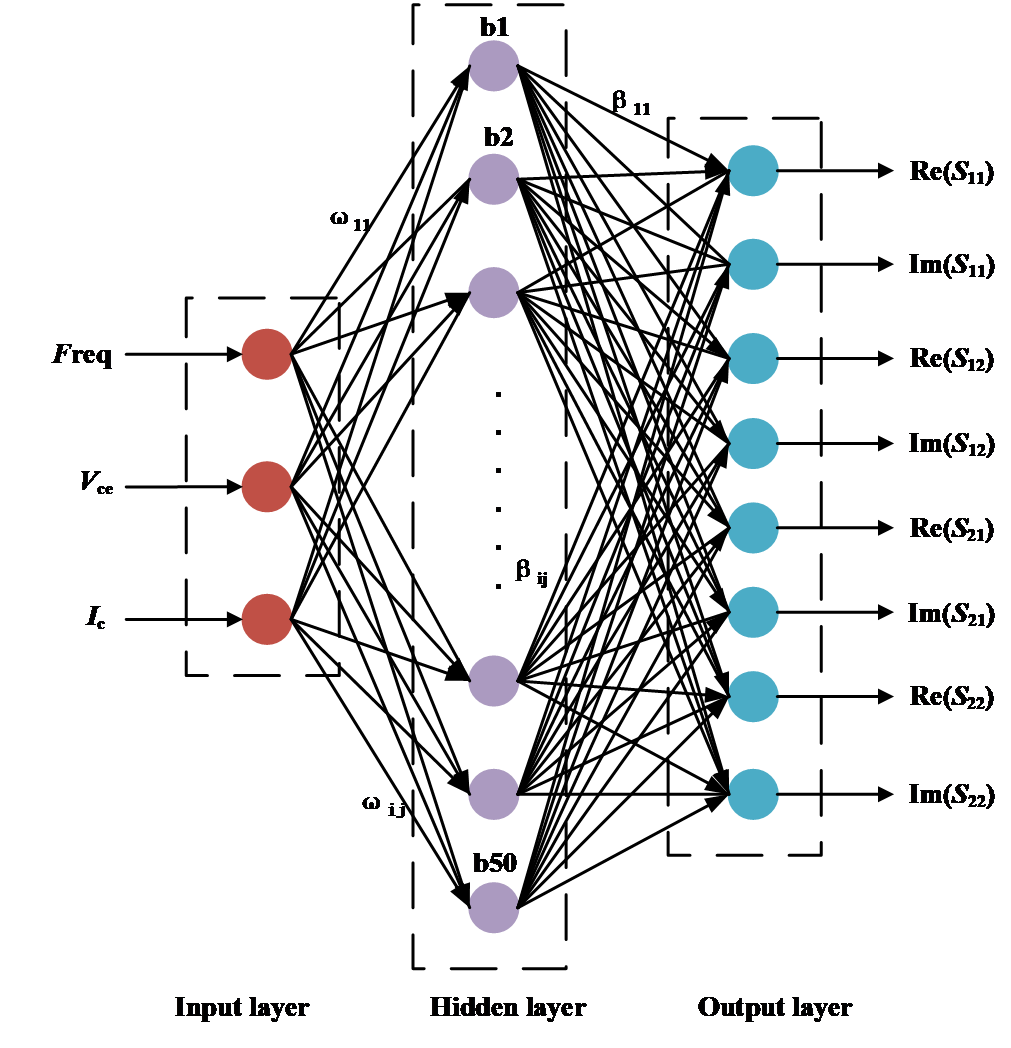##### Fig. 5. Flowchart of PSO-ELM algorithm.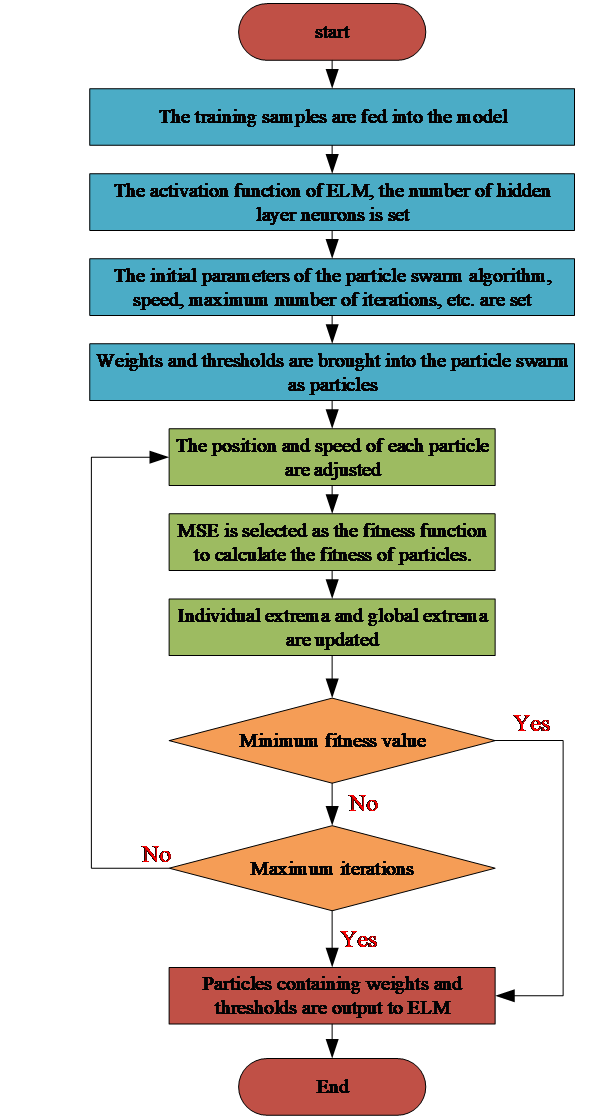##### Fig. 6. The measured and simulated results of I$_{\mathrm{c}}$-V$_{\mathrm{ce}}$ characteristics.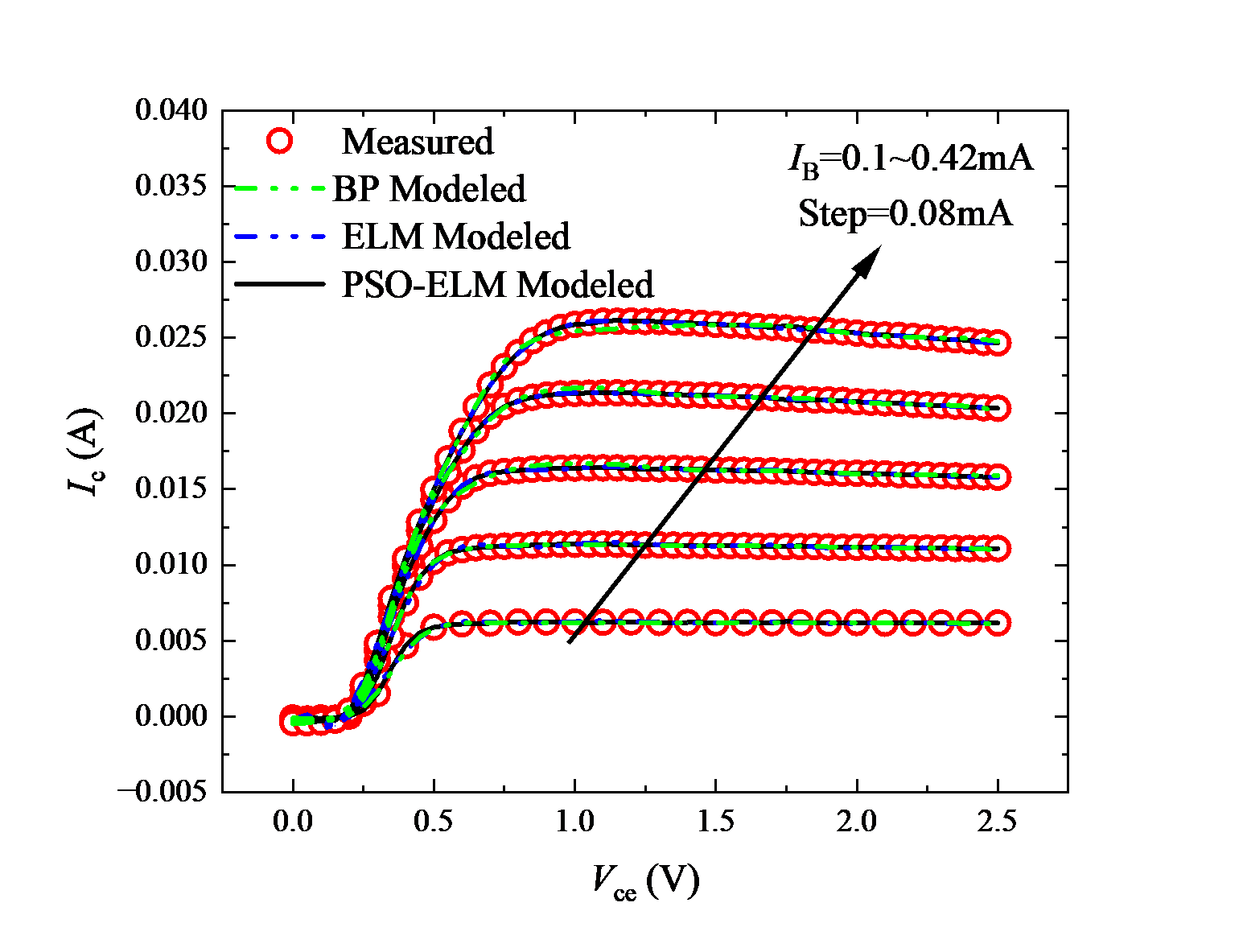##### Fig. 7. Measured and simulated curves of S-parameters at I$_{\mathrm{c}}$=20~mA and V$_{\mathrm{ce}}$=1.7 V.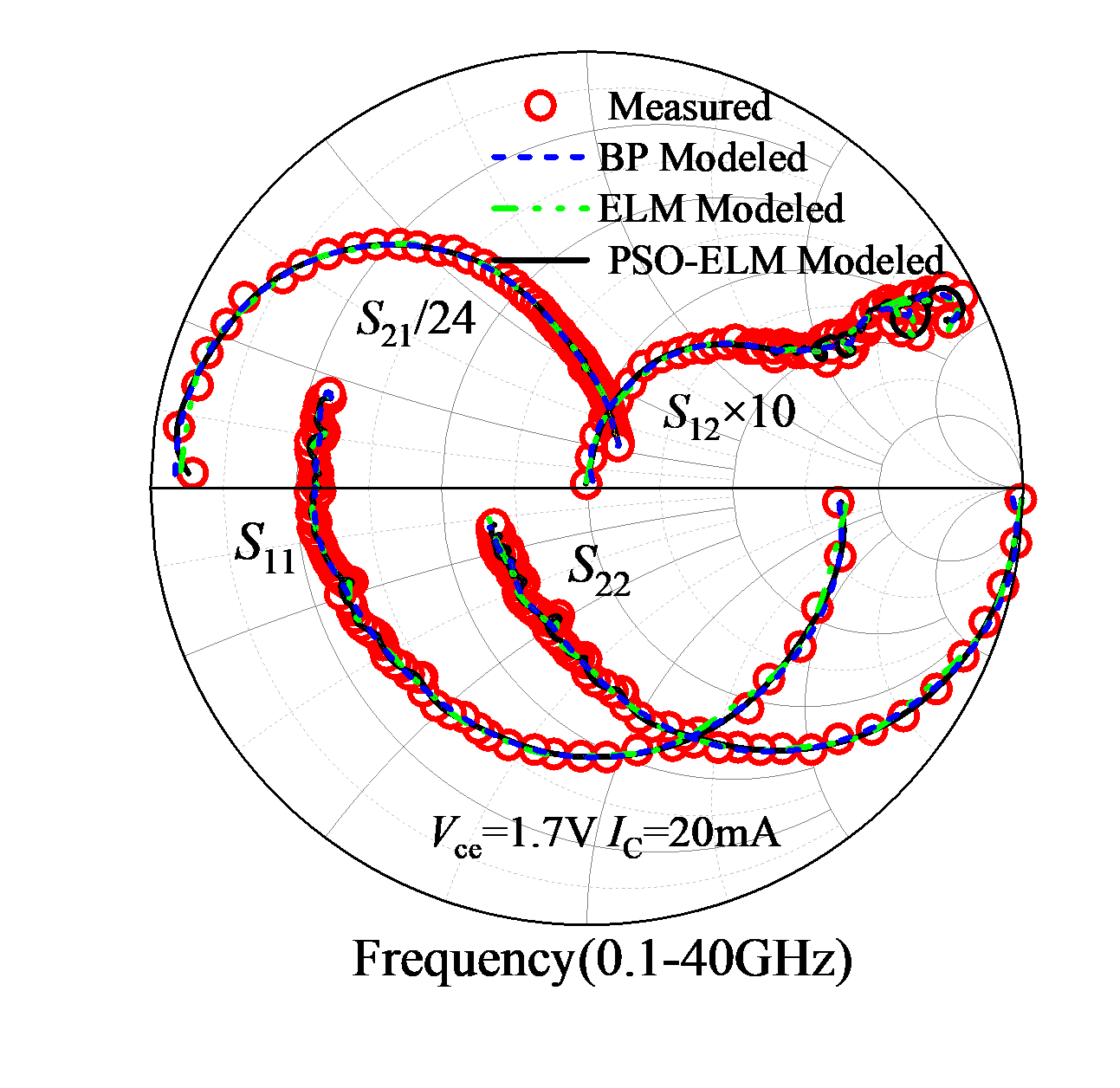##### Fig. 8. BP model training error under different bias conditions.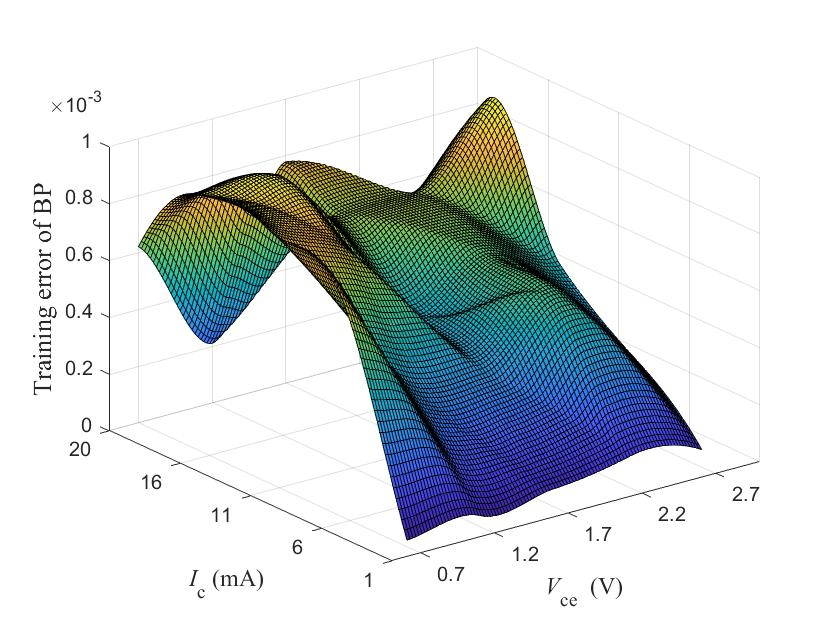##### Fig. 9. ELM model training error under different bias conditions.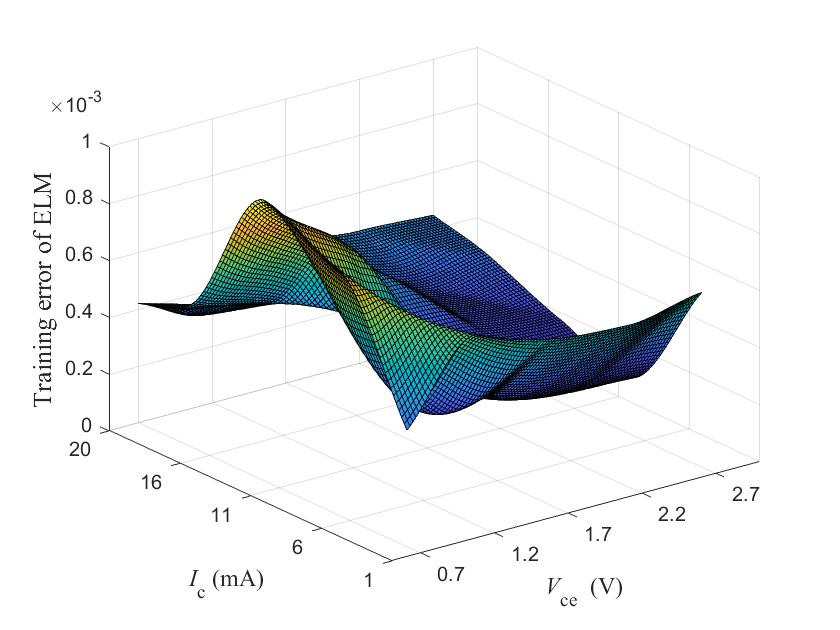##### Fig. 10. PSO-ELM model training error under different bias conditions.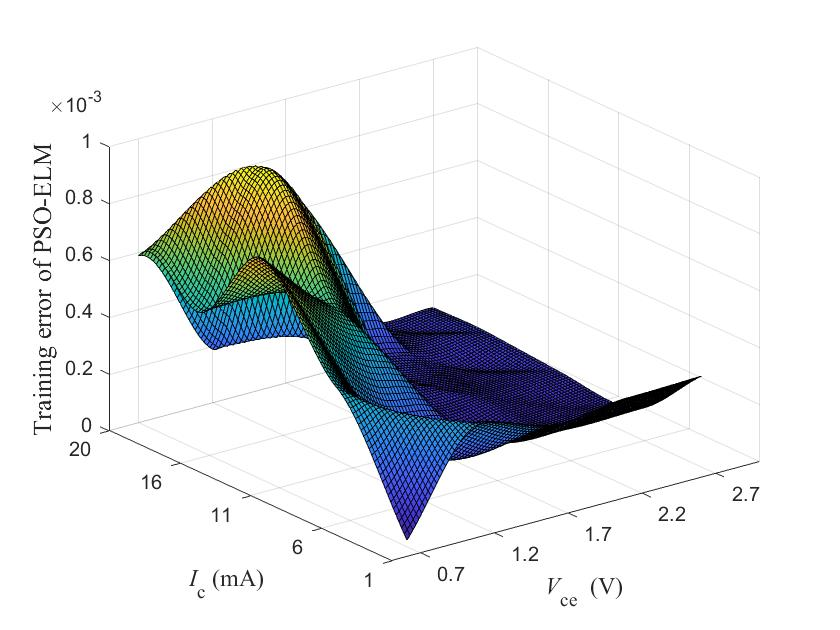##### Fig. 11. BP model test error under different bias conditions.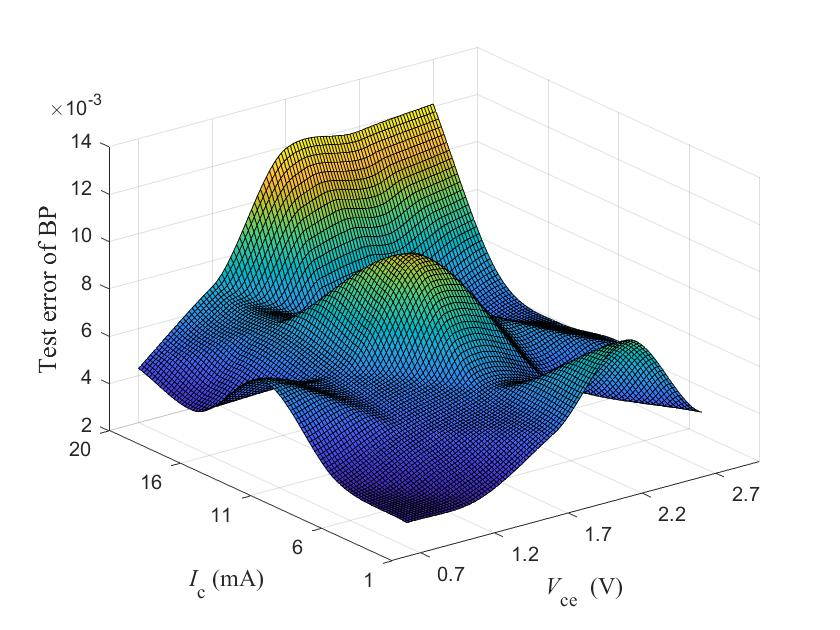##### Fig. 12. ELM model test error under different bias conditions.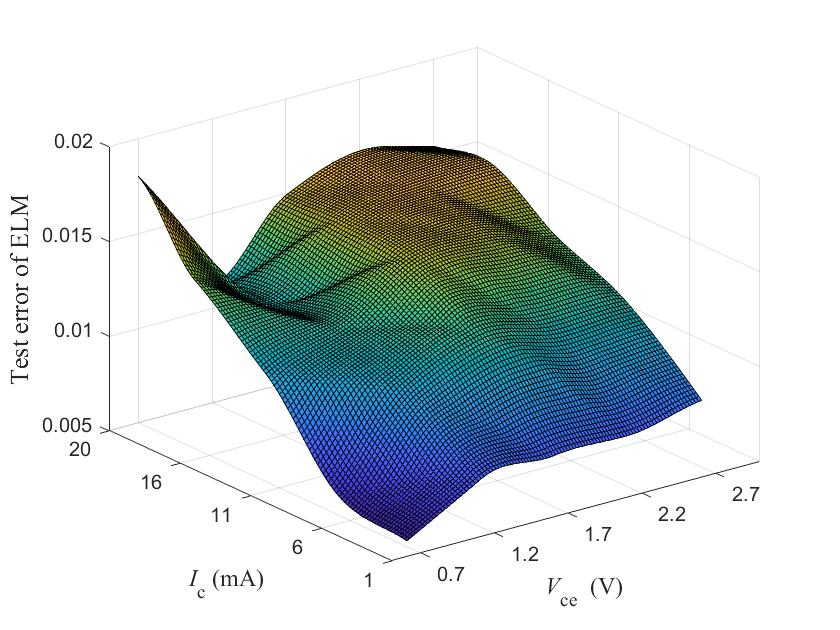##### Fig. 13. PSO-ELM model test error under different bias conditions.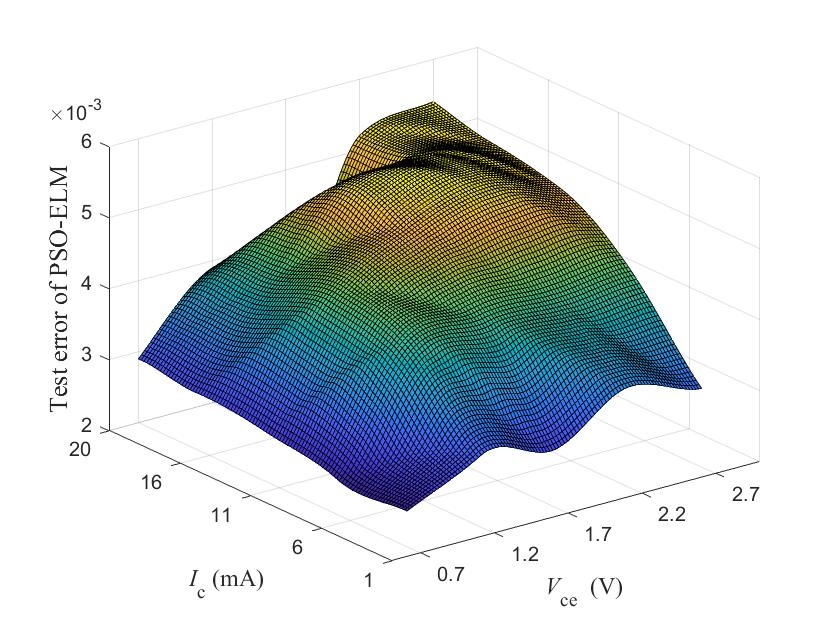## V. CONCLUSION

In order to avoid the influence of nonlinear factors on the establishment of HBT small-signal model, a more accurate device model is established. In this paper, a neural network modeling method based on PSO-ELM is proposed to model HBT small-signal model. The neural network model can directly model device characteristics without deriving extraction equations of model elements parameters. The experiments indicate that PSO algorithm can optimize the input weights and hidden layer thresholds of ELM, which avoids the possible ill-conditioned output of ELM. Through the comparison and error analysis of the ELM and PSO-ELM modeling results for a 1 ${\mu}$m${\times}$15 ${\mu}$m InP HBT, it is proved that the PSO-ELM model greatly improves the accuracy and generalization performance of the ELM model.

## ACKNOWLEDGMENTS

This work was supported by the National Natural Science Foundation of China (Grant No: 61804046), the Foundation of Department of Science and Technology of Henan Province (Grant No. 222102210172, 222102210207), and the Foundation of He’nan Educational Committee (Grant No. 21A510002).

## References

1
Arias-Purdue A., Rowell P., Urteaga M., et al. , 2020, A 120-mW, Q-band InP HBT Power Amplifier with 46% Peak PAE, 2020 IEEE/MTT-S International Microwave Symposium (IMS), pp. 1291-12942
Liu Z., Sharma T., Chappidi C. R., et al. , 2021, A 42-62 GHz Transformer-Based Broadband mm-Wave InP PA With Second-Harmonic Waveform Engineering and Enhanced Linearity, IEEE Trans. Microw. Theory Tech., Vol. 69, No. 1, pp. 756-7733
Zhang Y., Chen Y., Li Y., et al. , 2020., Modeling technology of InP heterojunction bipolar transistor for THz integrated circuit, Int. J. Numer. Model. Electron. Netw. Devices Fields, Vol. 33, No. 3, pp. e25794
Jin X., Müller M., Sakalas P., et al. , 2021, Advanced SiGe:C HBTs at Cryogenic Temperatures and Their Compact Modeling With Temperature Scaling, IEEE J. Explor. Solid-State Comput. Devices Circuits, Vol. 7, No. 2, pp. 175-1835
Nidhin K., Pande S., Yadav S., et al. , 2020, An Efficient Thermal Model for Multifinger SiGe HBTs Under Real Operating Condition, IEEE Trans. Electron Devices, Vol. 67, No. 11, pp. 5069-50756
Sun X., Zhang X., Sun Y., 2020., Thermal characterization and design of GaAs HBT with heat source drifting effects under large current operating condition, Microelectron. J., Vol. 100, pp. 1047797
Zhang A., Gao J., 2021, An Improved Nonlinear Model for Millimeter-Wave InP HBT Including DC/AC Dispersion Effects, IEEE Microw. Wirel. Compon. Lett., Vol. 31, No. 5, pp. 465-4688
Sun Y., Liu Z., Li X., et al. , 2019, Distributed Small-Signal Equivalent Circuit Model and Parameter Extraction for SiGe HBT, IEEE Access, Vol. 7, pp. 5865-58739
Johansen T. K., Leblanc R., Poulain J., et al. , 2016, Direct Extraction of InP/GaAsSb/InP DHBT Equivalent-Circuit Elements From S-Parameters Measured at Cut-Off and Normal Bias Conditions, IEEE Trans. Microw. Theory Tech., Vol. 64, No. 1, pp. 115-12410
Zhang J., Liu M., Wang J., et al. , 2021, An analytic method for parameter extraction of InP HBTs small-signal model, Circuit World11
Qi J., Lyu H., Zhang Y., et al. , 2020, An improved direct extraction method for InP HBT small-signal model, J. Infrared Millim. Waves, Vol. 39, No. 11, pp. 295-29912
Zhang A., Gao J., 2018, A new method for determination of PAD capacitances for GaAs HBTs based on scalable small signal equivalent circuit model, Solid-State Electron., Vol. 150, No. DEC., pp. 45-5013
Zhang J., Zhang L., Liu M., et al. , 2020, Systematic and Rigorous Extraction Procedure for InP HBT π-type Small-signal Model Parameters, J. Semicond. Technol. Sci., Vol. 20, No. 4, pp. 372-38014
Hu C., Horng J. B., Tseng H. C., 2011, Figures-of-merit genetic extraction for InGaAs lasers, SiGe low-noise amplifiers, ZnSe/Ge/GaAs HBTs, Int. J. Numer. Model. Electron. Netw. Devices Fields15
Munshi K., Vempada P., Prasad S., et al. , 2003, Small signal and large signal modeling of HBT’s using neural networks, 6th International Conference on Telecommunications in Modern Satellite, Cable and Broadcasting Service TELSIKS 2003., Vol. 2, pp. 565-56816
Wu H., Cheng Q., Yan S., et al. , 2015, Transistor Model Building for a Microwave Power Heterojunction Bipolar Transistor, IEEE Microw. Mag., Vol. 16, No. 2, pp. 85-9217
Han X., Tan H., Liu W., et al. , 2022., Modeling of heterojunction bipolar transistors based on novel Wiener-type dynamic neural network, Int. J. RF Microw. Comput.-Aided Eng., Vol. 32, No. 4, pp. e2307218
Jarndal A., Husain S., Hashmi M., et al. , 2021, Large-Signal Modeling of GaN HEMTs Using Hybrid GA-ANN, PSO-SVR, GPR-Based Approaches, IEEE J. Electron Devices Soc., Vol. 9, pp. 195-20819
Hussein A. S., Jarndal A. H., 2018, Reliable Hybrid Small-Signal Modeling of GaN HEMTs Based on Particle-Swarm-Optimization, IEEE Trans. Comput.-Aided Des. Integr. Circuits Syst., Vol. 37, No. 9, pp. 1816-182420
Huang G. B., 2014, An Insight into Extreme Learning Machines: Random Neurons, Random Features and Kernels, Cogn. Comput., Vol. 6, No. 3, pp. 376-39021
Anupam S., Pani P., 2020, Flood forecasting using a hybrid extreme learning machine-particle swarm optimization algorithm (ELM-PSO) model, Model. Earth Syst. Environ., Vol. 6, No. 4, pp. 1-722
Yao L., Xu S., Xiao Y., et al. , 2022, Fault Identification of Lithium-Ion Battery Pack for Electric Vehicle Based on GA Optimized ELM Neural Network, IEEE Access, Vol. 10, pp. 15007-1502223
Melchor-Leal J. M., Cantoral-Ceballos J. A., 2021, Force profile characterization for thermostatic bimetal using extreme learning machine, IEEE Lat. Am. Trans., Vol. 19, No. 02, pp. 208-21624
Tahir G. A., Chu K. L., 2020, An Open-Ended Continual Learning for Food Recognition Using Class Incremental Extreme Learning Machines, IEEE Access25
Sheinman B., Wasige E., Rudolph M., et al. , 2002, A peeling algorithm for extraction of the HBT small-signal equivalent circuit, IEEE Transactions on Microwave Theory and Techniques, Vol. 50, No. 12, pp. 2804-281026
Bataller-Mompeán M., Martínez-Villena J. M., Rosado-Muñoz A., et al. , 2016, Support Tool for the Combined Software/Hardware Design of On-Chip ELM Training for SLFF Neural Networks, IEEE Trans. Ind. Inform., Vol. 12, No. 3, pp. 1114-112327
Wang Y., Cao F., Yuan Y., 2011, A study on effectiveness of extreme learning machine, Neurocomputing, Vol. 74, No. 16, pp. 2483-249028
Gu R., Shen F., Huang Y., et al. , 2013, A parallel computing platform for training large scale neural networks, 2013 IEEE International Conference on Big Data, pp. 376-38429
Liu X., Lin S., Fang J., et al. , 2015, Is Extreme Learning Machine Feasible? A Theoretical Assessment (Part I), IEEE Trans. Neural Netw. Learn. Syst., Vol. 26, No. 1, pp. 7-2030
Eberhart R., Kennedy J., 1995, A new optimizer using particle swarm theory, MHS'95. Proceedings of the Sixth International Symposium on Micro Machine and Human Science, pp. 39-4331
Cai W., Yang J., Yu Y., et al. , 2020, PSO-ELM: A Hybrid Learning Model for Short-Term Traffic Flow Forecasting, IEEE Access, Vol. 8, pp. 6505-651432
Wang S., Zhang J., Liu M., et al. , 2022, Large-Signal Behavior Modeling of GaN P-HEMT Based on GA-ELM Neural Network, Circuits Syst. Signal Process., Vol. 41, No. 4, pp. 1834-184733
Lu H. Y., Cheng W., Chen G., et al. , 2013, Direct Extraction Method of InP HBT Small-Signal Model, Applied Mechanics and Materials, vol. 347-350, Trans Tech Publications Ltd, pp. 1621-162434
Gao J. J., Li X. P., Wang H., et al , 2006, An approach to determine small-signal model parameters for InP-based heterojunction bipolar transistors, IEEE Transactions on Semiconductor Manufacturing, Vol. 19, No. 1, pp. 138-14535
Bousnina S., Mandeville P., Kouki A. B., et al , 2002, Direct parameter-extraction method for HBT small-signal model, in IEEE Transactions on Microwave Theory and Techniques, Vol. 50, No. 2, pp. 529-536Zhang Jincan was born in Xingtai, China, in 1985. He received the M.S. degree in Xi’an University of Technology, Xi’an, China, in 2010. He received the Ph.D. degree in XiDian University, Xi’an, China, in June 2014. Now He is an associate professor in Henan University of Science and Technology, Luoyang, China. His research is focused on modeling of HBTs and design of very high speed integrated circuit.

Fan Yunhang was born in Zhoukou, China, in 1998. He received a bachelor's degree from Henan University of Science and Technology in 2021. His research is focused on modeling of GaAs P-HEMT and design of very high speed integrated circuit.

Liu Min was born in Baoding, China, in 1984. She received the Ph.D. degree in XiDian University, Xi’an, China, in June 2016. Now she is a lecturer in Henan university of science and technology, Luoyang, China. Her research is focused on modeling of HBTs and design of integrated circuits.

Wang Jinchan was born in Luoyang, China, in 1980. She received the Ph. D. degree in Southeast University, Nanjing, China, in June 2009. Now she is an associate professor in Henan University of Science and Technology, Luoyang, China. Her research is focused on semiconductor materials and devices.

Zhang Liwen was born in 1980. She obtained her B.E and M.S. degree in Physics from Zhengzhou University, Zhengzhou, from 1997 to 2004; then received her Ph.D. degree in Atomic and Molecular Physics at Wuhan Institute of Physics and Mathematics, Chinese Academy of Sciences, Wuhan, in 2008. She is currently a Professor in Henan University of Science and Technology. Her major field is modeling and simulation in advanced packaging development.